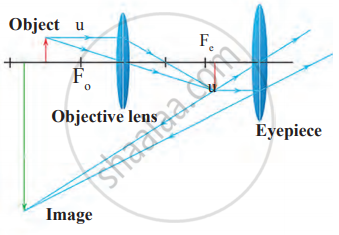# Observe the given figure and answer the following questions. 1. Where is the above type of lens construction used? 2. What type of image is formed by an objective lens? - Science and Technology 1

Short Note

Observe the given figure and answer the following questions.1. Where is the above type of lens construction used?
2. What type of image is formed by an objective lens?
3. What happens instead of placing at Fo if the object is placed in between O and Fo?

#### Solution

1. The above type of lens construction is used in a compound microscope.
2. The image formed by the objective is real, inverted, and formed at a distance within the focal length of the eyepiece.
3. If the object is placed in between O and Fo, a virtual image will be formed on the same side of the objective as that of the object. As a result, compound microscope will not be able to operate as expected.
Concept: Convex Lens
Is there an error in this question or solution?

Share